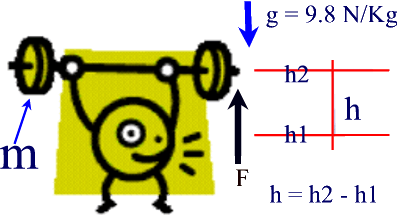#### Work Done When Lifting Objects

A weight lifter lifts the weights havig a mass m, at rest on a stand that is at a height h1, above the floor to a second level at a height h2 above ground, against gravity.The dfference between  h1  and  h is the net height (h) he lifts the weights against gravity.
To calculate the work done in lifting the weights at height h can be found by adaptong our basic work equation

Therefore
Work = Force x distance
= Force of Gravity x heoght
= Fg x h

Recall that the force of gravity Fg = m x g

Therefore, the work done in lifting an object against gravity can be calculated using the equation

W = m x g x h

Example

What is the work done by an elevator on 5 people (800 Kg) inside the elevator, if they all get off on the 4th. floor (12 m above the ground)?

Solution:

Given:
m = 800 Kg, g = 9.8 N/Kg, h = 12 m

Find:  W

W = m x g x h
= 800 Kg x 9.8 N/Kg x 12 m
= 94080 Nm
= 94 080 J

Therefore, the work done in lifting an object against gravity can be calculated using the equation  W = m x g x h

Example 2:

What is the work done by an elevator on 5 people (800 Kg) inside the elevator, if they all get off on the 4th. floor (12 m above the ground)?

Solution:

Given:
m = 800 Kg, g = 9.8 N/Kg, h = 12 m

Find:  W

W = m x g x h
= 800 Kg x 9.8 N/Kg x 12 m
= 94080 Nm
= 94 080 J